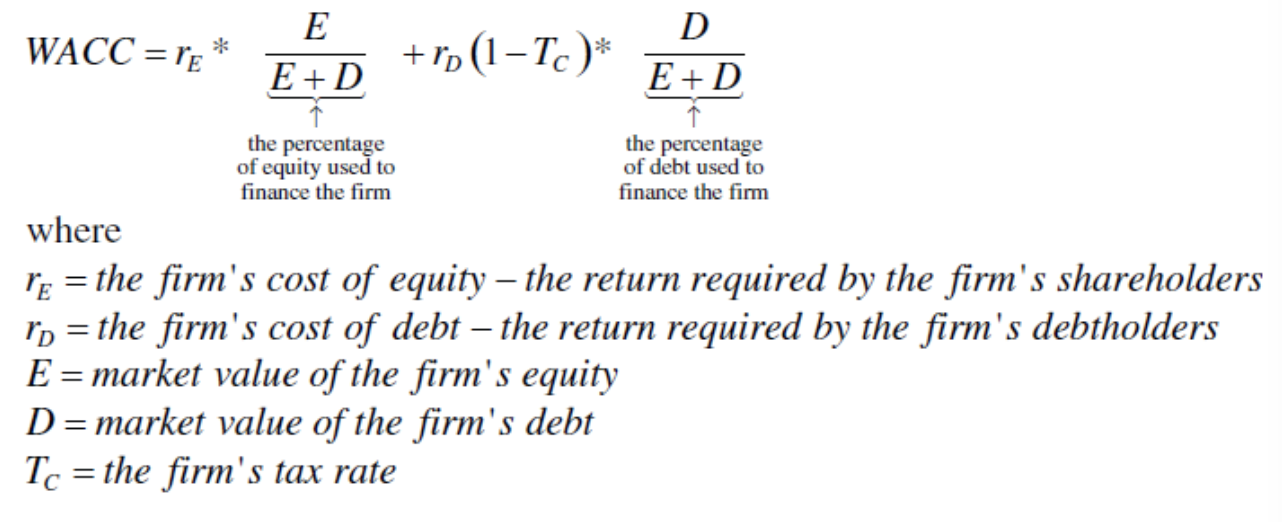### Chapter 3: Interest and Equivalence

tl;dr money at different times should be valued at different ratios, based on interest rates that compound

``````
↑ F=172,220
1965          |
--------------- 2013
|
↓ P=18

F = P(F|P,i,N), F|P mean F given P
F = 18(1+i)^48
solving for i, we get 21% annual ROR over 50 years, or Return on Revenue``````

Nominal is the stated interest rate, agnostic of compounding period (sometimes called APR)

• 12% compounded monthly refers to the nominal rate, but is actually Effectively 12.68%

Annuity starts not at payment period 0 but at 1.

• P1 = A(P given A, i, N)
• multiplying (1+i)n is (F/P,i,N)
• multiply by 1/(1+i)^n is (P/F,i,N)

### Chapter 4: Equivalence of Repeated Cash Flows

For uniform series over time, to calculate future amount:For uniform series calculating its present value (considering compounding interest):### Chapter 5: Present Worth Analysis

One way of comparing things is with present worth analysis

• independent alternatives are the cash flows of each alternative not relating
• mutually exclusive alternatives choose only one out of many
• present worth analysis is evaluating mutually exlcusive alternatives at the present time
• net present worth = PW(benefit) - PW(cost)

### Chapter 8: Risk

Average, Sqr, Varp, Stdevp, Frequency, Count, Ln

Three risk characteristics of financial assets

• Horizon, how long it takes to get money
• Safety is there guarantee?
• Liquidity how easy it is to sell the asset

Ex-ante is the expected return of an investment

• on a bond, we already know what to expect, so you can simply calculate ex-ante

Ex-post is a measure of past performance

• actual return realized upon sale of the asset
• will be the same as ex-ante if you sell at bond maturity date

### Chapter 6: Choosing a discount rate

We used net present value and internal rate of return

• for discounting,
• Weighted average cost of capital (WACC) is the funding cost for many corporate projects### Chapter 15: Minimum Attractive Rate of Return (MARR)

Assumed interested rate was given so far, but in practice we compute different rates or MARR

• closed end mutual funds IPO on stock market and there's a fixed number
• open end mutual funds have an unlimited number of units, but must have a reserve for "redemption"
• redemption has a fee